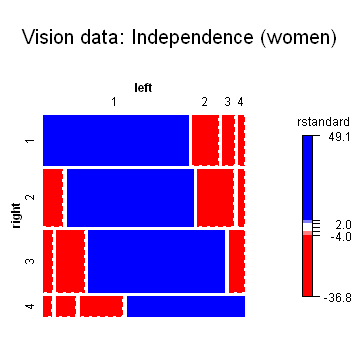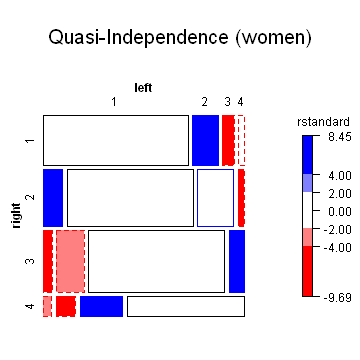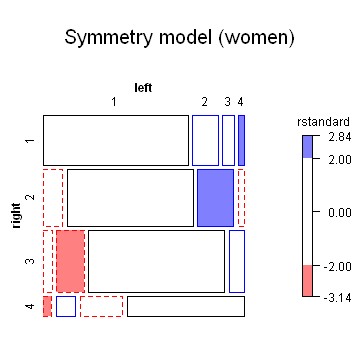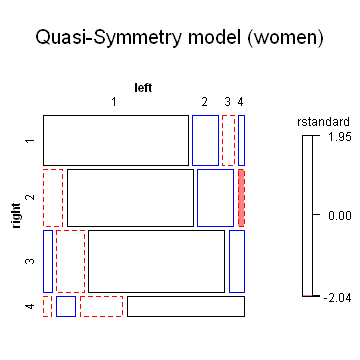# Output from vision-quasi.R

```library(vcdExtra)
library(gnm)
women <- subset(VisualAcuity, gender == "female", select = -gender)

indep <- glm(Freq ~ right + left, data = women, family = poisson)
mosaic(indep, residuals_type = "rstandard", gp = shading_Friendly, main = "Vision data: Independence (women)")
```
```## Warning: no formula provided, assuming ~right + left
``````# Quasi-independence: ignore the diagonal cells by fitting them exactly

quasi.indep <- glm(Freq ~ right + left + Diag(right, left), data = women, family = poisson)
mosaic(quasi.indep, residuals_type = "rstandard", gp = shading_Friendly, main = "Quasi-Independence (women)")
```
```## Warning: no formula provided, assuming ~right + left
``````# Symmetry: test F[i,j] = F[j,i].  Note that the model does not include the 'main'
# effects of right and left, so assumes marginal homogeneity

symmetry <- glm(Freq ~ Symm(right, left), data = women, family = poisson)
mosaic(symmetry, residuals_type = "rstandard", gp = shading_Friendly, main = "Symmetry model (women)")
```
```## Warning: no formula provided, assuming ~right + left
``````# Quasi-symmetry: allow different marginal frequencies for left and right

quasi.symm <- glm(Freq ~ right + left + Symm(right, left), data = women, family = poisson)
mosaic(quasi.symm, residuals_type = "rstandard", gp = shading_Friendly, main = "Quasi-Symmetry model (women)")
```
```## Warning: no formula provided, assuming ~right + left
``````# model comparisons: for *nested* models
anova(indep, quasi.indep, quasi.symm, test = "Chisq")
```
```## Analysis of Deviance Table
##
## Model 1: Freq ~ right + left
## Model 2: Freq ~ right + left + Diag(right, left)
## Model 3: Freq ~ right + left + Symm(right, left)
##   Resid. Df Resid. Dev Df Deviance Pr(>Chi)
## 1         9       6672
## 2         5        199  4     6472   <2e-16 ***
## 3         3          7  2      192   <2e-16 ***
## ---
## Signif. codes:  0 '***' 0.001 '**' 0.01 '*' 0.05 '.' 0.1 ' ' 1
```
```anova(symmetry, quasi.symm, test = "Chisq")
```
```## Analysis of Deviance Table
##
## Model 1: Freq ~ Symm(right, left)
## Model 2: Freq ~ right + left + Symm(right, left)
##   Resid. Df Resid. Dev Df Deviance Pr(>Chi)
## 1         6      19.25
## 2         3       7.27  3       12   0.0075 **
## ---
## Signif. codes:  0 '***' 0.001 '**' 0.01 '*' 0.05 '.' 0.1 ' ' 1
```

Generated with `R version 2.15.1 (2012-06-22)` using the R package knitr (version `0.8.4`) on `Wed Sep 26 09:01:50 2012`.